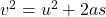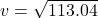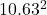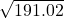## A 51.0 kg box, starting from rest, is pulled across a floor with a constant horizontal force of 240 N. For the first 12.0 m the floor is fri

Question

A 51.0 kg box, starting from rest, is pulled across a floor with a constant horizontal force of 240 N. For the first 12.0 m the floor is frictionless, and for the next 10.5 m the coefficient of friction is 0.21. What is the final speed of the crate after being pulled these 20.5 meters?

in progress 0
1 month 2021-08-12T14:03:21+00:00 1 Answers 0 views 0

The final speed of the crate after being pulled these 20.5 meters is 13.82 m/s

Explanation:

I’ll assume that the correct question is

A 51.0 kg box, starting from rest, is pulled across a floor with a constant horizontal force of 240 N. For the first 12.0 m the floor is frictionless, and for the next 10.5 m the coefficient of friction is 0.21. What is the final speed of the crate after being pulled these 22.5 meters?

mass of box = 51 kg

for the first 12 m, it is pulled with a constant force of 240 N

The acceleration of the box for this first 12 m will be

from F = ma

a = F/m

where F is the pulling force

m is the mass of the box

a is the acceleration of the box

a = 240/51 = 4.71 m/s^2

Since the body started from rest, the initial velocity u = 0

applying Newton’s equation of motion to find the final velocity at the end of the first 12 m, we havewhere v is the final velocity

u is the initial velocity which is zero

a is the acceleration of 4.71 m/s^2

s is the distance covered which is 12 m

substituting value, we have= 0 + 2(4.71 x 12)= 113.04= 10.63 m/s

For the final 10.5 m, coefficient of friction is 0.21

from  f = μF

where f is the frictional force,

μ is the coefficient of friction = 0.21

and F is the pulling force of the box 240 N

f = 0.21 x 240 = 50.4 N

Net force on the box = 240 – 50.4 = 189.6 N

acceleration = F/m = 189.6/51 = 3.72 m/s^2

Applying newton’s equation of motionu is initial velocity, which in this case =  10.63 m/s

a = 3.72 m/s^2

s = 10.5 m

v = ?

substituting values, we have=+ 2(3.72 x 10.5)= 112.9 + 78.12

v  == 13.82 m/s